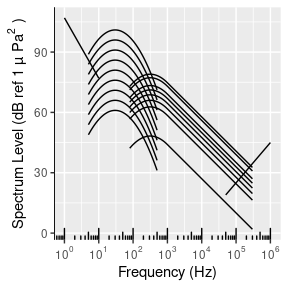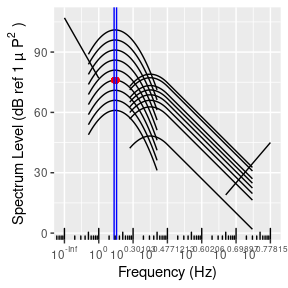# The Sound Stops with the Passive Sonar Equation

The bioSNR package is an open-source SONAR equation calculator. The calculator is capable of handling simple to intermediate level acoustic problems associated with bioacoustics and passive acoustic monitoring (PAM) systems.

This document gives quick examples of bioSNR’s capabilities with the commonly utilized passive sonar equation.

#Stable - Install package from CRAN
install.packages("bioSNR")

#Unstable - Install package from Github repository
devtools::install_github("MattyD797/bioSNR")

#Attach package namespace to active libraries in Rstudio
library(bioSNR)

### The Passive Sonar Equation

The passive sonar equation describes the relationship, or sound-to-noise ratio (SNR), of an underwater sound from a source to receiver. This equation is useful for determining detection ranges of recording units, as well as acoustic active space, that is the distance from the sourcce over which the signal’s amplitude renains above the detection threshold of potential listeners (Marten and Marlet, 1977). It terms of bioacoustics, the equation can be outlined as follows:

$SL-TL-(NL-PG)\geq DT$

• $$SL$$ is the source level of the signal of interest, as measured in dBs.
• $$TL$$ is the transmission loss or propagation loss (e.g., absorption, reflection, and diffusion).
• $$NL$$ is the noise level or background ambient noise in the recorder’s local environment.
• $$PG$$ is the processing gain or directivity index which reduces the noise level.
• $$DT$$ is the detection threshold, or the additional dBs the signal of interest must achieve above ambient noise conditions in order to be detected by the receiver.

### Source Level

Before we start applying the passive sonal equation, let’s further decompose its parts. The $$SL$$ is typically measured 1 meter away from the source (~3 wavelengths). This also means that $$SL$$ is the ratio between the transmitted intensity from the source and the reference intensity. Refer to the vignette decibels for a refresher on dBs or the introduction vignette for more information on intensity, power, and pressure.

If we take a simulated sound source, such as a clown fish called Nemo, we can better understand the physics! Let’s say for simplicity that Nemo emits a perfect omni-directional sound. We refer to this uniform prorogation of sound power as spherical spreading. Referring to the definition of $$SL$$, we can form this equation:

$SL=10log\frac{I_s}{I_{ref}}$

• $$I_s$$ is the intensity of the transmitted signal from 1 meter or approximately 3 wavelengths away.
• $$I_{ref}$$ is the intensity of a sound with a root mean square pressure of $$1\;\mu Pa$$. Note that in bioacoustics pressure is typically referred to as the root-mean-square pressure because of the sinusoidal nature of sound waves.
• $$SL$$ is the source level in $$dB \:re\: 1\;\mu Pa$$, but also maintains the intensity of a $$1\;\mu Pa$$ signal.

By referring to the equation for intensity, we can easily identify the relationship between pressure and intensity ($$I = \frac{P_{rms}^2}{z}$$). Just in case, a similar relationship between intensity and power can also be expressed as $$I = \frac{P}{4\pi r^2}$$. The $$SL$$ can then be expressed in power or pressure as well:

$SL=10log_{10}\frac{I_s}{I_{ref}} = 10log_{10}\frac{P}{4\pi I_{ref}} = 10log_{10}P-10log_{10}(4\pi I_{ref}) = 10log_{10}P+170.8$

$...= 10log_{10}\frac{P_{rms}^2}{\rho c I_{ref}} = 10log_{10}P_{rms}^2-10log_{10}(\rho c * 6.7*10^{-19})$

• Where $$I_{ref}$$ is equal to $$1 \mu Pa$$ (as documented in the decibels vignette: $$1 \mu Pa = 6.7*10^{-19}\: \frac{W}{m^2}$$)

### Transmission Loss

Nemo’s sound will eventually soften as the sound wave moves through the water. This propagation loss is caused by resistance of water particles to move and the properties of the water itself. The exact definition is the ratio of sound intensity at 1 m from a source to the sound intensity at distance $$r$$. Thus, transmission loss can be represented as the following:

$TL = 10log\frac{I_s}{I_sR}$

$$TL$$ is the most complicated parameter in the passive sonar equation, but in an idealized ocean environment transmission loss has two components: 1) geometric spreading and 2) absorption of the sound as it propagates. Geometric spreading (1) is the primary contributor to transmission loss. As the signal of interest’s energy geometrically spreads over a larger area, the intensity of the sound lowers over the increasing distance. Absorption (2) involves the absorption coefficient which we provide a function to calculate in the introduction vignette. We discuss in greater depth what factors are considered in calculating this measurement.

Geometric spreading is characterized as either spherical or cylindrical. Spreading begins as spherical, but as the signal of interest continues to spread across larger disances, the surface of the ocean contrains the rounded top of the sphere, forcing it into a cylindrical shape. So spherical spreading transitions into cylindrical once it hits the limiting factor of the ocean’s depth. The transition from spherical to cylindrical is rarely uniform, but for the sake of idealized equations, we will maintain a transition range of half the ocean’s depth at the site of the sound source. Below is the geometric transmission loss for spherical and then for cylindrical (before and after transition range):

$TL_{spherical}=20log_{10}r$

$TL_{cylindrical}=10log_{10}r + 10log_{10}r_{trans}$

• $$r$$ is the propagation distance.
• $$r_{trans}$$ is transition range or half the depth.

The absorption coefficient computed using absorptionWater() is calculated via the viscous absorption, boric acid relaxation, and magnesium sulfate relaxation process. While these equations are explained well in other literature, these explanations are beyond our need of understanding absorption in the context of bioacoustics, so we will not repeat them here. When we do factor teh abssorption coefficient into geometrical spreading, the formula takes on the following form:

$TL=TL_{spherical | cylindrical}(r)+\alpha r$

• $$\alpha$$ is the absorption coefficient calculated using the function bioSNR function absorptionWater().

### Noise Level and Processing Gain

Noise level ($$NL$$) is the ratio between the average background noise intensity and reference intensity that is the same as used in the $$SL$$ (typically $$I_{ref} = 6.7*10^{-19}\: \frac{W}{m^2}$$). While the equation is the same as $$SL$$ we can use a Wenz curve (Wenz 1962) to estimate spectrum levels, an approach adapted into the function specLvlGraph(). Below is the empirical Wenz curve for odontocetes (toothed whales), that describe oceanic ambient noise as a function of frequency:$$NL$$ can then be found via the following formula:

$NL=\text{SpectrumLvl} + 10log_{10}(\text{Bandwidth})$

• $$Bandwidth$$ is equal to the difference between the maximum to minimum frequency of the measured frequency band.

An array of recorders can reduce overall noise level. By combining their independently measurements of the soundscape the signal-to-noise ratio is increased, which is referred to as propagation gain ($$PG$$) or array gain ($$AG$$). This is typically specified on the recording array of use. A variety of formulas can also calculate the $$PG$$ value called (e.g., ‘beamforming’) but this computation is currenltly beyond the scope of this package. $$PG$$ can be assumed to be 1 $$dB\: re\: 1 \mu Pa$$ unless otherwise stated or known.

#### Examples

Each example will utilize the following information:

Nemo suddenly turned into a blue whale (Balaenoptera musculus intermedia) and produced a call near a Rockhopper recording unit. The call was made at 195 $$dB\: re\: 1 \mu Pa$$ at 1 meter and measured between [28,33] Hz frequency.

1. Using a Wenz curve, what would be the noise level in the frequency band of interest, considering noise from moderate shipping, a sea state of 1, and a wind speed of 10 mph?
specLvlGraph(c(28,33), ship=4,seaState = 1, wSpeed = 10, boolR = T)[]
#> The noise level (NL) is: 82.9896990174959 dB ref 1 µP• The $$NL$$ is 82.9897 $$dB\: re \: 1\mu Pa$$.
1. Given the above $$NL$$, we want to find the detection range of the call. We can neglect absorption at this distance (a=0) in the function rmax(). Given we want a $$DT$$ of 10 $$dB\: re \: 1\mu Pa$$, we can calculate $$NL$$ by finding the propagation distance in the formula for $$TL$$ and rewriting the passive sonar equation: $$TL=SL-NL-DT$$. We can further break down the equation of $$TL_{cylindrical}$$:

$TL_{cylindrical}=10log_{10}r + 10log_{10}r_{trans}+\alpha r$

Into:

$10log_{10}r + 10log_{10}r_{trans} + \alpha r = SL-NL-DT$

#Source Level
SL <- 195
#Noise Level - from above
NL <- 82.9897
#Detection Threshold
DT <- 10
#Transition Range <- depth/2
TR <- 2500

rmax(sl=SL,nl=NL,dt=DT,d=5000, xaxis=10000000)
#>  6354626

The call has a detection range of 6354626 meters. Nemo has some chords!

Wenz, Gordon M. 1962. “Acoustic Ambient Noise in the Ocean: Spectra and Sources.” The Journal of the Acoustical Society of America. Acoustical Society of America.